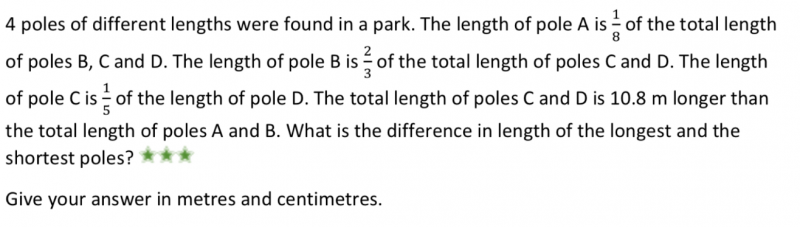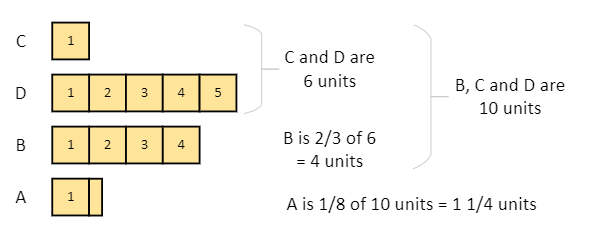# QuestionHelp plz```We get the above block diagram from facts in the questions:
- C is 1/5 of D. So C + D is 6 units.
- B is 2/3 of C and D. So B is 2/3 x 6 = 4 units
- B, C and D is 6 + 4 = 10 units
- A is 1/8 of B, C and D. So A is 10/8 = 1 1/4 units

A and B is 5 1/4 units. C and D is 6 units.
Difference is 6 - 5 1/4 = 3/4 units = 10.8 m.
So each unit is 10.8 x 4/3 = 14.4 m.

Longest pole is D. Shortest pole is C.
Difference is 4 units = 4 x 14.4m = 57.6m = 57m 60cm.
```

0 Replies 2 Likes

Let D be 5u

D     [u][u][u][u][u]

C     [u]                               (1/5 of D)

B = (2/3) (5u+u) = 4u

A = (1/8)( 6u + 4u) = (10/8)u = (5/4)u = (1 ¼  u

C + D (6u)    is 10.8m longer than A + B (5 ¼  u

6u5 ¼  u = 10.8

¾ u = 10.8

u =14.4

Longest = D = 5u = 5(14.4) = 72

Shortest = C = u = 14.4

Difference between longest and shortest pole = 72 – 14.4 = 57.6m = 57m 60 cm

0 Replies 1 Like